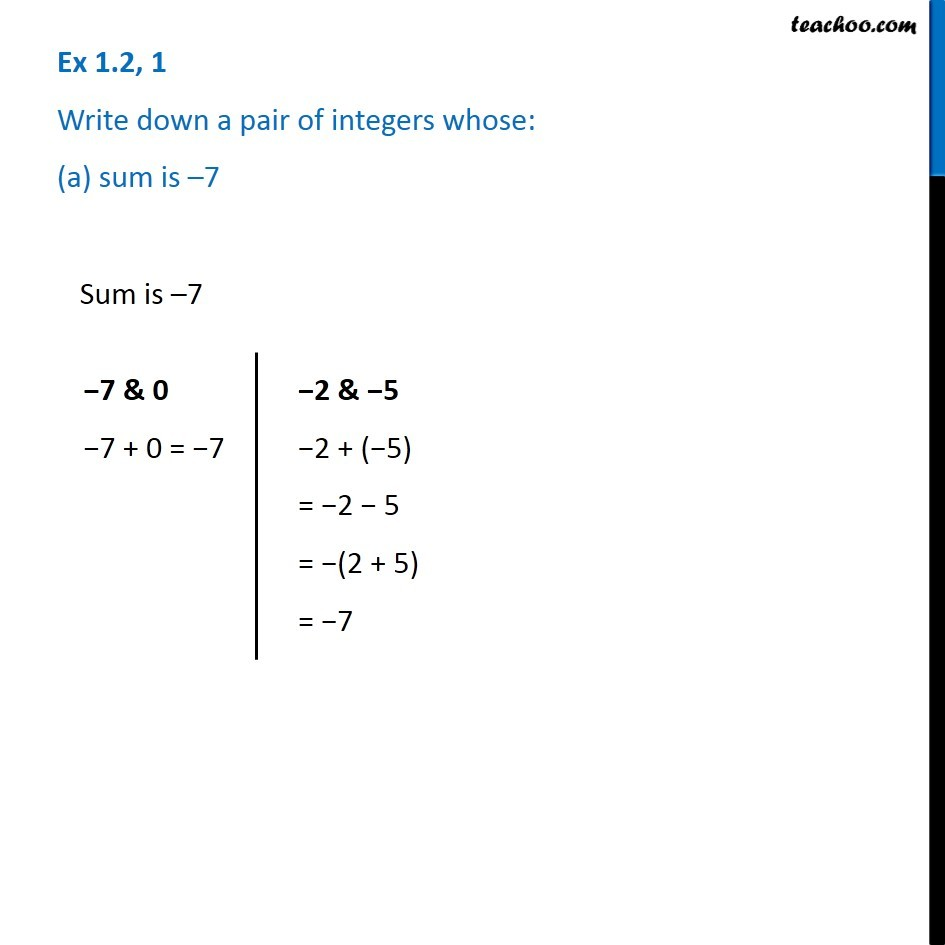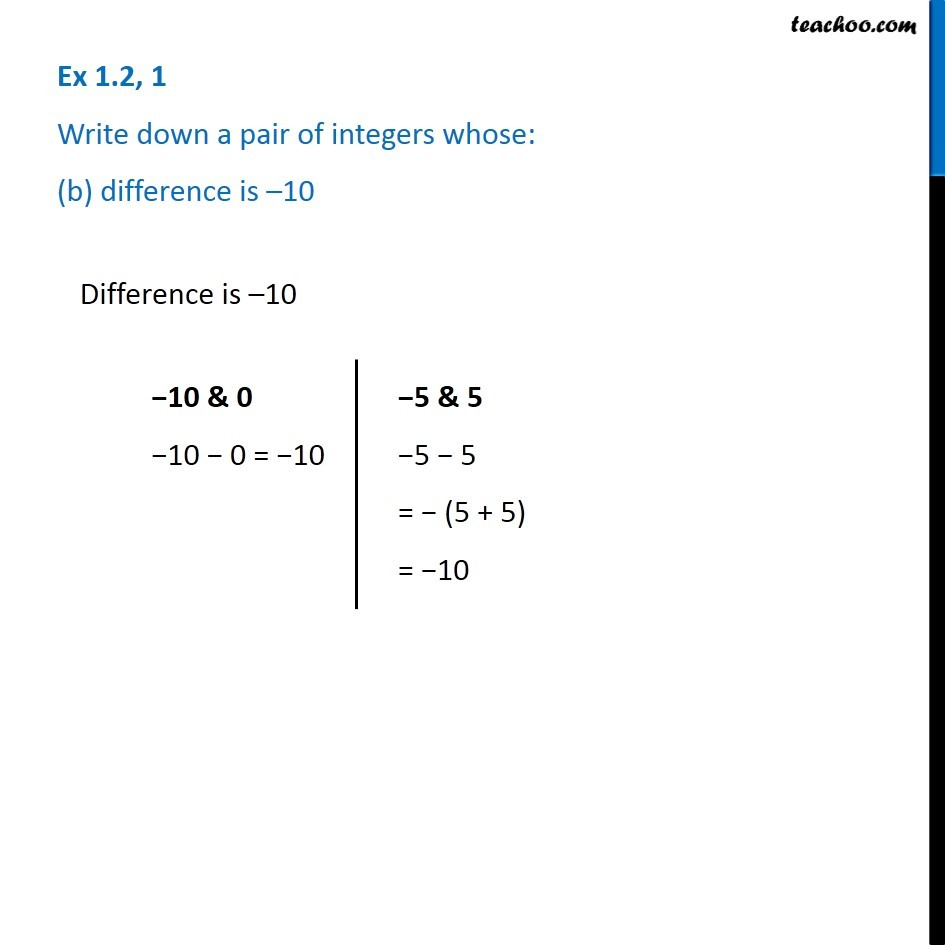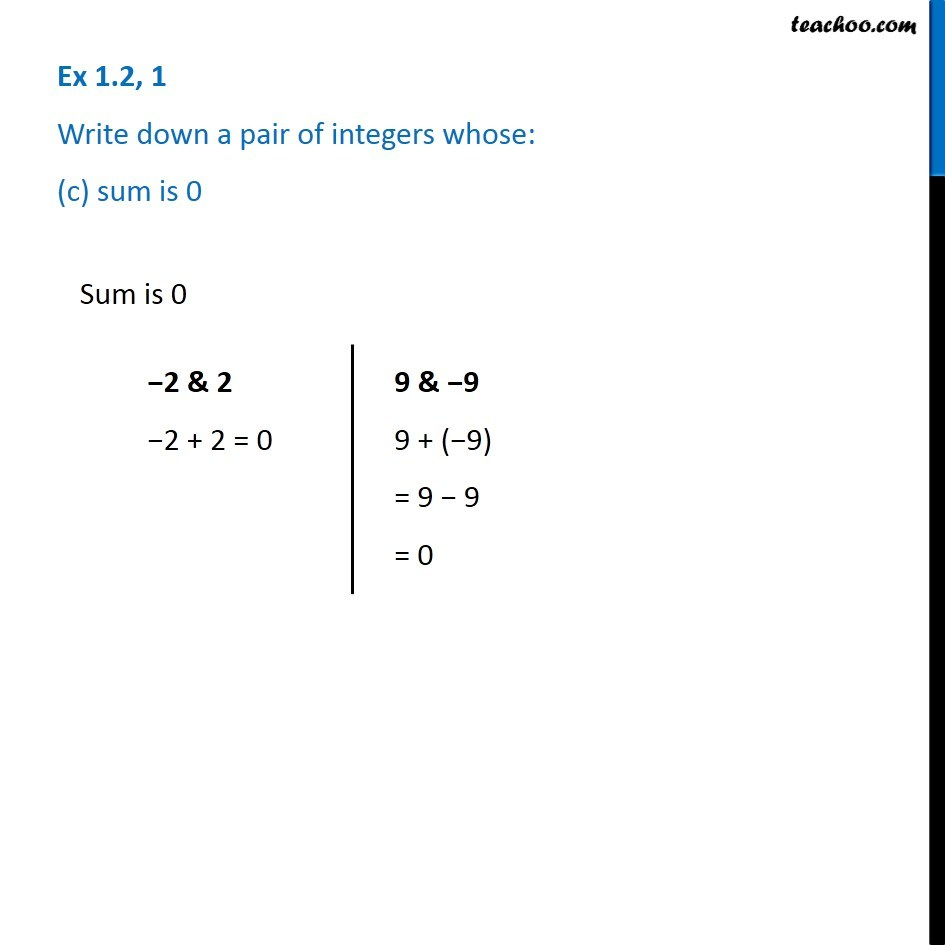Subscribe to our Youtube Channel - https://you.tube/teachoo

1. Chapter 1 Class 7 Integers
2. Serial order wise
3. Ex 1.2

Transcript

Ex 1.2, 1 Write down a pair of integers whose: (a) sum is 7 Sum is 7 7 & 0 7 + 0 = 7 2 & 5 2 + ( 5) = 2 5 = (2 + 5) = 7 Ex 1.2, 1 Write down a pair of integers whose: (b) difference is 10 Difference is 10 10 & 0 10 0 = 10 5 & 5 5 5 = (5 + 5) = 10 Ex 1.2, 1 Write down a pair of integers whose: (c) sum is 0 Sum is 0 2 & 2 2 + 2 = 0 9 & 9 9 + ( 9) = 9 9 = 0

Ex 1.2

Chapter 1 Class 7 Integers
Serial order wise